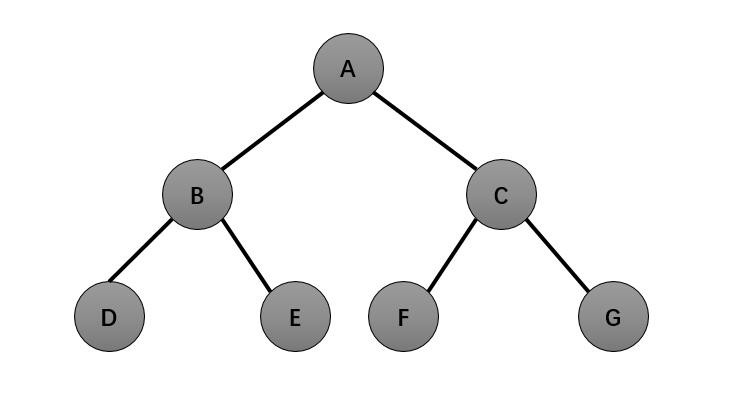# 分治法求最值问题，典型的错误示范及正确的代码

笔者在进行分治法学习和跟同学讨论时发现一个比较有意思的错误，在此分享给大家。

我们知道，分治法的核心思想时将待处理对象先分后治，而且当待处理对象基本有序时分治法将退化为冒泡算法。

下面我们看一个有意思的错误示范（伪代码）：

void MaxMin(A[l..r],Max,Min)
{
if(r==l)
{
if(A>Max) Max=A[l];
else if(A<Min) Min=[l];
}
else{
MaxMin(A[l,(l+r)/2],Max1,Min1); //递归解决前一部分
MaxMin(A[(l+r/)2+1..r],Max2,Min2); //递归解决后一部分
}

}

上述程序确实能求出最值，但是值得我们注意的是它虽然将待处理数组分开了，但却并不是分治，换句话说它其实根本没用分治法的算法，而是冒泡排序。我们把分治法分开后的结构理解为二叉树，它处理的思路是将最值分别与叶子结点做比较，无论待排序处理对象如何变化，它的时间复杂度都和冒泡排序完全一样（其实这就是冒泡排序的递归式）。下面我们再用二叉树的结构通过图文结合来理解分治法的思想：D、E、F、G是分后所得，其求解最值的思想是每一个节点是以其为根节点的树的最优解，比如B所得的最值的是DE比较后产生的，C所得的最值是FG比较后产生的，而A所得的值又是BC比较后产生的。说到这里如果还有读者不能清楚的话，我再做一个简单的比喻：在上述的二叉树中，如果用冒泡排序求最大值，MAX依次DEFG比较（比较次数是四次），分治法将DE比较得B，FG比较得C，BC比较得A（比较次数是三次）。

void MaxMin(A[l..r],Max,Min)
{
if(r==l)
{//只有一个元素时
Max=A[l];
Min=[l];
}
else
{
if(r－l==1)  //有两个元素时
{
if (A[l]<=A[r]){
Max=A[r];
Min=A[l];
}
else{
Max=A[l];
Min=A[r];
}
}
else{//r－l>1
MaxMin(A[l,(l+r)/2],Max1,Min1); //递归解决前一部分
MaxMin(A[(l+r/)2+1..r],Max2,Min2); //递归解决后一部分
}
if (Max1<Max2)
Max= Max2;//从两部分的两个最大值中选择大值
if (Min2<Min1)
Min=Min2; //从两部分的两个最小值中选择小值
}

}
这是笔者从求最值的角度对分治法探讨，所得难免不够客观，欢迎读者留言探讨。